# Class 10 Maths Problems for Application of trigonometry

Question 1.
Two poles of equal heights are standing opposite to each other on either side of the road which is 80m wide. From a point between them on the road the angles of elevation of the top of the poles are 60°and 30°.find the height of the poles and the distances of the point from the poles.
Question 2.
A man sitting at a height of 20m on a tall tree on a small island in the middle of the river observes two poles directly opposite to each other on the two banks of the river and in line with the foot of tree. If the angles depression of the feet of the poles from a point at which the man is sitting on the tree on either side of the river are 60° and 30° respectively. Find the width of the river.
Question 3.
A vertical tower stands on a horizontal plane and is surmounted by a flag- staff of height 7m. From a point on the plane, the angle of elevation of the bottom of the flag- staff is 30° and that of the top of the flag- staff is 45°. Find the height of the tower.
Question 4.
A man on the deck of a ship is 10m above the water level. He observes that the angle of elevation of the top of a cliff is 45o and the angle of depression of the base is 30° calculate the distance of the cliff from the ship and the height of the cliff.
Question 5.
There are two temples, one on each bank of a river, just opposite to each other. One temple is 50m high. From the top of this temple, the angle of depression of the top and the foot of the other temple are 30° and 60°respectively. Find the width of the river and the height of the other temple.
Question 6.
An aero plane flying horizontally 1km above the ground is observed at an elevation of 60°. After 10 seconds, its elevation is observed to be 30°. Find the speed of the aero plane in km/hr.
Question 7.
A tree standing on a horizontal plane leaning towards east. At two points situated at distances $a$ and $b$ exactly due west on it, the angles of elevation of the top are respectively $\alpha$ and $\beta$.Prove that the height of the top from the ground is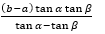Question 8.
The angle of elevation of the top of a vertical tower PQ from a point X on the ground is 60°. At a point Y, 40m vertically above X, the angle of elevation of the top is 45° Calculate the height of the tower.
Question 9.
If the angle of elevation of a cloud from a point h metre above a lake is $\alpha$ and the angle of depression of its reflection in the lake be b, prove that the distance of the cloud from the point of observation is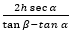Question 10.
From an aeroplane vertically above a straight horizontal road, the angles of depression of two consecutive mile stones on opposite sides of the aeroplane are observed to be $\alpha$ and $\beta$. Show that the height in miles of aeroplane above the road is given by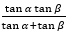Question 11.
A ladder rests against a wall at an angle $\alpha$ to the horizontal. Its foot is pulled away from the wall through a distance , so that its slides a distance b down the wall making an angle $\beta$ with the horizontal. Show that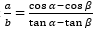Question 12.
A boy is standing on the ground and flying a kite with 100m of string at an elevation of 30°. Another boy is standing on the roof of a 10 m high building and is flying his kite at an elevation of 45°. Both the boys are on opposite sides of both the kites. Find the length of the string that the second boy must have so that the two kites meet.
Question 13.
Two boats approach a light house in mid- sea from opposite directions. The angles of elevation of the top of the light house from two boats are 30° and 45° respectively. If the distance between two boats is 100m, find the height of the light house.
Question 14.
From the top of a light house, the angles of depression of two ships on the opposite sides of it are observed to be $\alpha$ and $\beta$. If the height of the light house be h meters and the line joining the ships passes through the foot of the light house, show that the distance between the ship is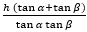metres
Question 15.
As observed from the top of a 150m tall light house, the angles of depression of two ships approaching it are 30° and °. If one ship is directly behind the other, find the distance between the two ships.

1. 20√3m,20m, 60m
2. 80/(√3)m
3. 9.56m
4. Distance = 10√3m, Height = 27.32m
5. 28.83m, 33.33m
6. 415.68km/hr
8. 94.64m
12. 40√2m
13. 50 (√3 – 1) m
15. 109.5m

Go back to Class 10 Main Page using below links

### Practice Question

Question 1 What is $1 - \sqrt {3}$ ?
A) Non terminating repeating
B) Non terminating non repeating
C) Terminating
D) None of the above
Question 2 The volume of the largest right circular cone that can be cut out from a cube of edge 4.2 cm is?
A) 19.4 cm3
B) 12 cm3
C) 78.6 cm3
D) 58.2 cm3
Question 3 The sum of the first three terms of an AP is 33. If the product of the first and the third term exceeds the second term by 29, the AP is ?
A) 2 ,21,11
B) 1,10,19
C) -1 ,8,17
D) 2 ,11,20